## Longer Differences and Smoothing¶

One way to reduce $\sigma_k/\sigma_R$ is to reduce noise in new cases by taking a longer difference or smoothing case counts in some other way. How does this affect the estimation and interpretation of $R_t$?

As in the first section, we start with the approximate recursive relation If we instead look at a longer difference, where $\overline {T_{t,L} e^{\gamma(R_{t,L} - 1)}}$ is some intermediate value in between the minimum and maximum of the ${ \frac{\tau(t-i)}{\tau(t-i-1)} e^{\gamma (R_{t-i} - 1)} }_{i=0}^{L-1}$.

If testing is constant over time, we can then obtain an interpretable $\overline{R_{t,L}}$ by using $k_{t,L} =\log(C(t)-C(t-L))$ and following the procedure above.

If testing varies with time, it becomes hard to separate testing rate changes from $R_t$ after taking long differnces.

Note

The same analysis can be applied to other smoothing operations, i.e. using in place of $C(t) - C(t-L)$. However, there’s something strange about smoothing $C_t$, and then extracting a smoothed component of it using the Kalman filter. The inference afterwards is suspect; we would essentially be estimating a kernel regression of $C_t$ on time, and using the estimated regression as though it’s known with certainty.

When would long differences reduce variance? Well if $\Delta C(t) = \Delta C^\ast(t) + \epsilon_t$ with $\epsilon_t$ indepenedent over time with mean $0$ and constant variance, then you would need $C^\ast(t) - C^\ast(t-L)$ to increase faster than linearly with $L$. This is true if $C^\ast$ is growing exponentially.

Alternatively, if $\epsilon_t$ is not independent over time, but negatively correlated (as seems likely), then variance can decrease with $L$. For example, if $\Delta C(t) = C^\ast(t) - C^\ast(t-\delta)$ with $\delta$ a random, independent increment with mean $1$, then variance will tend to decrease with $L$ regardless of $C^\ast(t)$.

## Results¶

Here, we will allow the initial and time varying mean of $R_{s,t}$ to depend on covariates.

reestimate=false
rlo=-1 #1 - eps(Float64)
rhi=1.2 #1+ eps(Float64)
K = size(X,2)
priors = (γ = truncated(Normal(1/7,1/7), 1/28, 1/1),
σR0 = truncated(Normal(1, 3), 0, Inf),
α0 = MvNormal(zeros(length(X0)), sqrt(10)), #truncated(Normal(1, 3), 0, Inf),
σR = truncated(Normal(0.25,1),0,Inf),
σk = truncated(Normal(0.1, 5), 0, Inf),
ρ = Uniform(rlo, rhi),
α = MvNormal(zeros(K), sqrt(10))
)
mdl = RT.RtRW(dlogk, X, X0, priors);
trans = as( (γ = asℝ₊, σR0 = asℝ₊, α0 = as(Array, length(X0)),
σR = asℝ₊, σk = asℝ₊, ρ=as(Real, rlo, rhi),
α = as(Array, K)) )
P = TransformedLogDensity(trans, mdl)

p0 = (γ = 1/7, σR0=1.0, α0 = zeros(length(X0)), σR=0.25, σk=2.0, ρ=1.0, α=zeros(K))
x0 = inverse(trans,p0)

2.090468 seconds (4.01 M allocations: 193.476 MiB, 9.03% gc time)

rng = MersenneTwister()
steps = 100
warmup=default_warmup_stages(local_optimization=nothing, #FindLocalOptimum(1e-6, 200),
stepsize_search=nothing,
init_steps=steps, middle_steps=steps,
terminating_steps=2*steps,  doubling_stages=4, M=Symmetric)
x0 = x0
if !isfile("rt7.jld2") || reestimate
res = DynamicHMC.mcmc_keep_warmup(rng, ∇P, 2000;initialization = (q = x0, ϵ=0.1),
reporter = LogProgressReport(nothing, 25, 15),
warmup_stages =warmup);
post = transform.(trans,res.inference.chain)
@save "rt7.jld2" post
end
p = post
vals = hcat([vcat([length(v)==1 ? v : vec(v) for v in values(p)]...) for p in post]...)'
vals = reshape(vals, size(vals)..., 1)
names = vcat([length(p[s])==1 ? String(s) : String.(s).*"[".*string.(1:length(p[s])).*"]" for s in keys(p)]...)
cc = MCMCChains.Chains(vals, names)
display(plot(cc))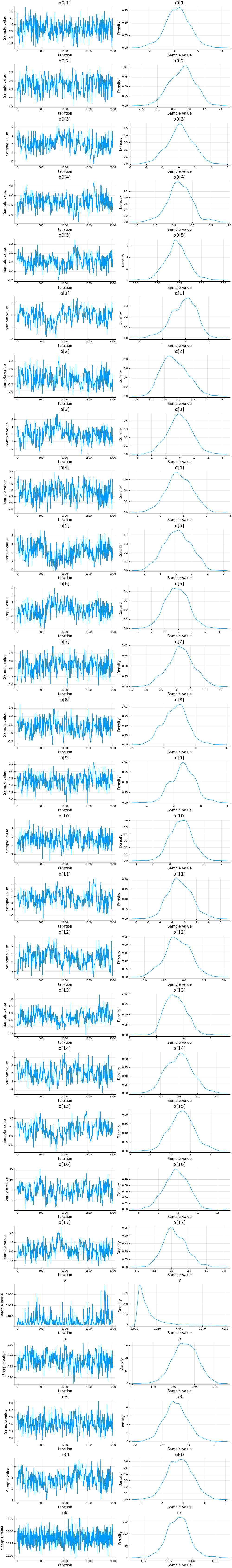display(describe(cc))

2-element Array{ChainDataFrame,1}

Summary Statistics
parameters     mean     std  naive_se    mcse       ess   r_hat
──────────  ───────  ──────  ────────  ──────  ────────  ──────
γ   0.0379  0.0025    0.0001  0.0003  108.5762  1.0094
σR0   2.8472  0.5901    0.0132  0.0656   48.7521  1.0343
α0   0.7643  2.5342    0.0567  0.1661  187.4434  1.0018
α0   0.7817  0.3966    0.0089  0.0296  171.7902  1.0026
α0   0.0789  0.7772    0.0174  0.0986   37.6326  1.0154
α0  -0.3337  0.3151    0.0070  0.0315   82.1813  1.0035
α0   0.2207  0.1280    0.0029  0.0147   47.5974  1.0322
σR   0.5057  0.0870    0.0019  0.0041  255.7555  1.0007
σk   0.1271  0.0024    0.0001  0.0001  446.0680  0.9998
ρ   0.9309  0.0116    0.0003  0.0010  107.4759  1.0163
α   1.8516  1.0681    0.0239  0.1446   25.1433  1.0501
α  -1.1438  0.4449    0.0099  0.0416  105.1060  1.0127
α  -0.0083  0.8520    0.0191  0.1048   44.8124  1.0340
α   0.8243  0.5430    0.0121  0.0493  124.4263  1.0032
α   0.0994  0.8315    0.0186  0.1093   31.5759  0.9995
α  -0.1280  0.8602    0.0192  0.1103   40.2397  0.9995
α   0.1713  0.4355    0.0097  0.0390  160.8671  1.0006
α  -0.6264  0.3775    0.0084  0.0300  124.0985  1.0104
α  -0.6664  0.4365    0.0098  0.0395   96.5867  1.0059
α  -0.3495  0.6313    0.0141  0.0483  173.2760  0.9999
α  -0.7192  1.9604    0.0438  0.2069   78.9574  1.0042
α  -0.7804  1.5990    0.0358  0.1891   39.9999  1.0244
α  -0.2912  0.3787    0.0085  0.0497   38.9951  1.0060
α   0.0375  1.7094    0.0382  0.2016   77.1582  1.0000
α   1.6999  1.8242    0.0408  0.2300   52.0837  1.0387
α   4.5390  2.9292    0.0655  0.3112   78.9740  1.0021
α   0.5028  1.6706    0.0374  0.2142   47.3735  1.0128

Quantiles
parameters     2.5%    25.0%    50.0%    75.0%    97.5%
──────────  ───────  ───────  ───────  ───────  ───────
γ   0.0358   0.0363   0.0370   0.0385   0.0447
σR0   1.7240   2.4285   2.8403   3.2445   4.0325
α0  -4.6969  -0.8651   0.8286   2.4189   5.8212
α0   0.0048   0.5012   0.8055   1.0475   1.5065
α0  -1.4842  -0.4245   0.0886   0.6008   1.5086
α0  -0.9854  -0.5299  -0.3391  -0.1320   0.3514
α0  -0.0282   0.1411   0.2189   0.2999   0.4784
σR   0.3405   0.4481   0.5033   0.5608   0.6871
σk   0.1224   0.1255   0.1272   0.1286   0.1318
ρ   0.9081   0.9232   0.9311   0.9394   0.9532
α  -0.4662   1.1087   1.9482   2.6229   3.7126
α  -1.8904  -1.4731  -1.1892  -0.8362  -0.2449
α  -1.6674  -0.5660  -0.0063   0.5603   1.6550
α  -0.2679   0.4651   0.8096   1.2006   1.8807
α  -1.5504  -0.4773   0.1007   0.7080   1.6550
α  -1.7526  -0.7365  -0.1388   0.4751   1.5934
α  -0.6846  -0.1063   0.1747   0.4540   1.0258
α  -1.3522  -0.8877  -0.6086  -0.3772   0.1172
α  -1.4709  -0.9545  -0.6563  -0.3671   0.2092
α  -1.5648  -0.7817  -0.3454   0.0693   0.8933
α  -4.1944  -2.0611  -0.8113   0.6417   3.3614
α  -4.0476  -1.7987  -0.7897   0.3959   2.3293
α  -0.9347  -0.5773  -0.3129  -0.0225   0.4680
α  -3.1091  -1.2218   0.0735   1.1604   3.4156
α  -1.7118   0.4843   1.7194   2.8932   5.6024
α  -1.3076   2.5101   4.5436   6.6371   9.8855
α  -2.5148  -0.6405   0.3466   1.6276   3.9682

display([1:length(x0vars)  x0vars])

5×2 Array{Any,2}:
1  :constant
2  :logpopdens
3  Symbol("Percent.Unemployed..2018.")
4  Symbol("Percent.living.under.the.federal.poverty.line..2018.")
5  Symbol("Percent.at.risk.for.serious.illness.due.to.COVID")

display([1:length(xvars) xvars])

17×2 Array{Any,2}:
1  :constant
2  Symbol("Stay.at.home..shelter.in.place")
3  Symbol("State.of.emergency")
4  Symbol("Date.closed.K.12.schools")
5  Symbol("Closed.gyms")
6  Symbol("Closed.movie.theaters")
7  Symbol("Closed.day.cares")
8  Symbol("Date.banned.visitors.to.nursing.homes")
10  Symbol("Closed.restaurants.except.take.out")
11  :retail_and_recreation_percent_change_from_baseline
12  :grocery_and_pharmacy_percent_change_from_baseline
13  :parks_percent_change_from_baseline
14  :transit_stations_percent_change_from_baseline
15  :workplaces_percent_change_from_baseline
16  :residential_percent_change_from_baseline

states = unique(sdf.state)
states_to_plot = ["New York", "New Jersey","Massachusetts","California",
"Georgia","Illinois","Michigan",
"Ohio","Wisconsin","Washington"]
S = length(states_to_plot)
figs = fill(plot(), S)
for (i,st) in enumerate(states_to_plot)
s = findfirst(states.==st)
figr = RT.plotpostr(dates[s],dlogk[s],post, X[s], X0[s])
l = @layout [a{.1h}; grid(1,1)]
figs[i] = plot(plot(annotation=(0.5,0.5, st), framestyle = :none),
plot(figr, ylim=(-1,10)), layout=l)
display(figs[i])
end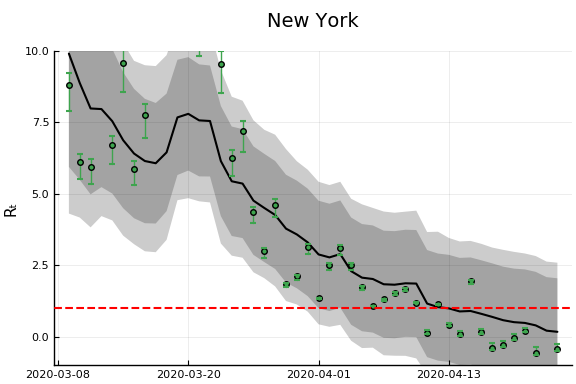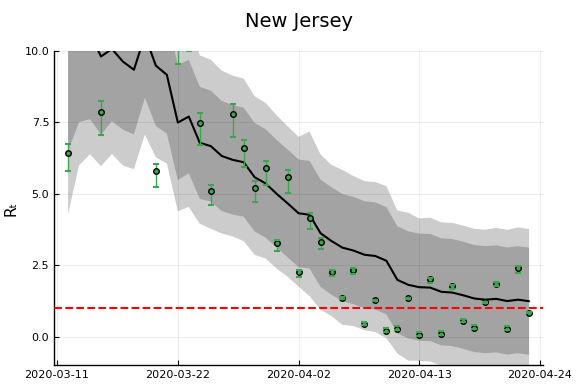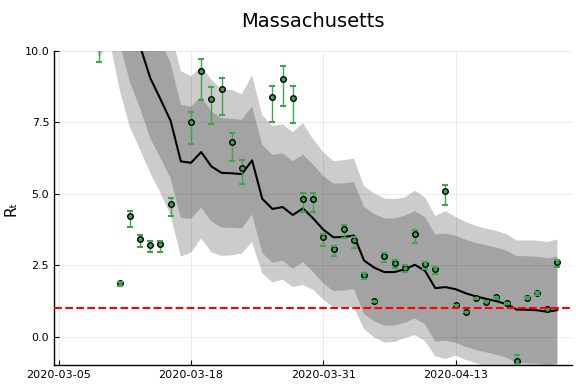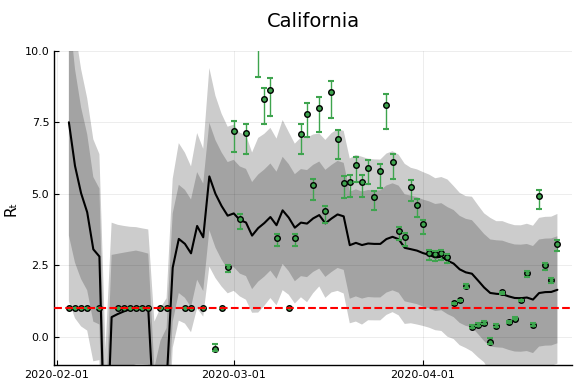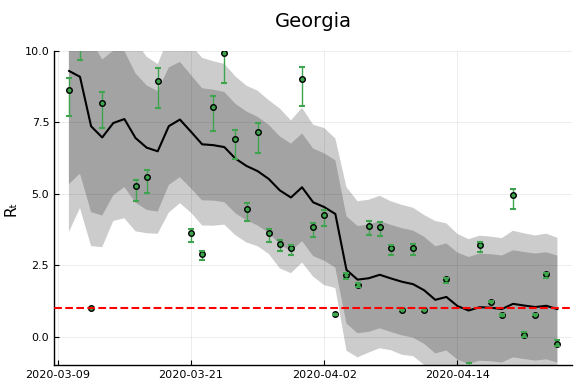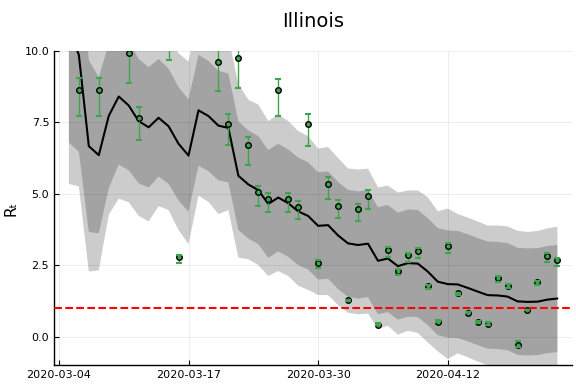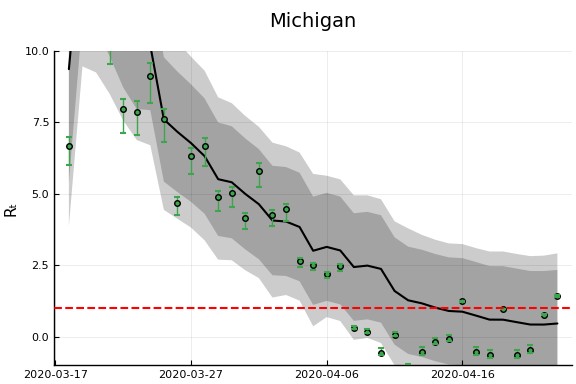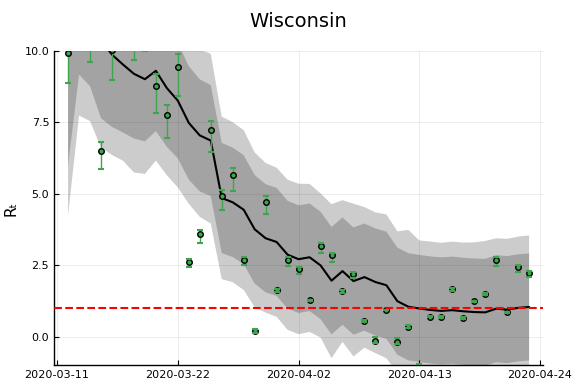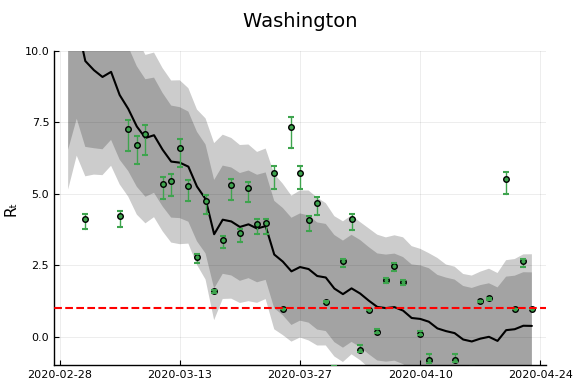# Another Derivation¶

An alternative (and easier) way to derive the same estimator will be described here. This approach will easily generalize to more complicated models, but let’s begin with the simplest SIR model with testing.

Then note that

and

which is the equation we have been using for estimation.

## Incorporating death¶

If we add deaths to the model,

then,

Other observable states could similarly be added to the model.

## Time delays¶

A drawback of the above approach is that it implies changes in $R_t$ show up in the derivatives of case and death numbers instantly. This is definitely not true. Instead consider a model where infections last $\ell$ days. After $\ell$ days, each infected person dies with probability $\pi$ and recovers otherwise. Then we have

Rearranging gives and

Note

These last two equations also hold in the model without time delay by setting $\ell=0$ and $\pi = \frac{p}{p+\gamma}$

Note

Random durations can be accomodated by replacing the shift by $\ell$ with a convolution.